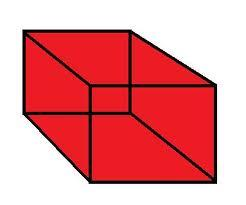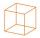# Special cube

Calculate the edge of cube, if its surface and its volume is numerically equal number.

a =  6

### Step-by-step explanation:Did you find an error or inaccuracy? Feel free to write us. Thank you!Tips to related online calculators
Do you have a linear equation or system of equations and looking for its solution? Or do you have a quadratic equation?

## Related math problems and questions:

• CubeCalculate the surface cube with edge 11 dm.
• CubeOne cube has an edge increased five times. How many times will larger it's surface area and volume?
• The cubeThe cube has a surface area of 486 m ^ 2. Calculate its volume.
• The cubeThe cube has a surface area of 216 dm2. Calculate: a) the content of one wall, b) edge length, c) cube volume.
• The cubeThe cube has a surface of 600 cm2. What is its volume?
• Cube V2SThe volume of the cube is 27 dm cubic. Calculate the surface of the cube.
• CubeThe sum of lengths of cube edges is 57 cm. What is its surface and volume?
• Cube edgeDetermine the edges of the cube when the surface is equal to 37.5 cm square.
• Length of the edgeFind the length of the edge of a cube with a cm2 surface and a volume in cm3 expressed by the same number.
• TerezaThe cube has an area of base 256 mm2. Calculate the edge length, volume, and area of its surface.
• Cube-shaped boxThe cube-shaped box is filled to the brim with 2 liters of milk. Calculate the edge and surface of the box.
• Cube surfce2volumeCalculate the volume of the cube if its surface is 150 cm2.
• Area of a cubeCalculate the surface area of a cube if its volume is equal to 729 cubic meters.
• Cube 6Volume of the cube is 216 cm3, calculate its surface area.
• Cube surface and volumeFind the surface of the cube with a volume of 27 dm3.
• Cube zoomHow many percents do we increase the volume and surface of the cube if we magnify its edge by 38 %?
• Cube surface and volumeThe surface of the cube is 500 cm2, how much cm3 will be its volume?